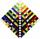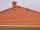# AP - simple

Determine the first nine elements of sequence if a10 = -1 and d = 4

Result

a9 =  -5
a8 =  -9
a7 =  -13
a6 =  -17
a5 =  -21
a4 =  -25
a3 =  -29
a2 =  -33
a1 =  -37

#### Solution:Leave us a comment of example and its solution (i.e. if it is still somewhat unclear...):

Showing 0 comments:Be the first to comment!## Next similar examples:

1. AP - simpleFind the first ten members of the sequence if a11 = 132, d = 3.
2. AS sequenceIn an arithmetic sequence is given the difference d = -3 and a71 = 455. a) Determine the value of a62 b) Determine the sum of 71 members.
3. Sequence 3Write the first 5 members of an arithmetic sequence: a4=-35, a11=-105.
4. Sequence 2Write the first 5 members of an arithmetic sequence a11=-14, d=-1
5. SequenceWrite the first 6 members of these sequence: a1 = 5 a2 = 7 an+2 = an+1 +2 an
6. SequenceWrite the first 7 members of an arithmetic sequence: a1=-3, d=6.
7. 6 termsFind the first six terms of the sequence. a1 = 7, an = an-1 + 6
8. SeatsSeats in the sport hall are organized so that each subsequent row has five more seats. First has 10 seats. How many seats are: a) in the eighth row b) in the eighteenth row
9. Sum of membersWhat is the sum of the first two members of the aritmetic progression if d = -4.3 and a3 = 7.5?
10. Nineteenth memberFind the nineteenth member of the arithmetic sequence: a1=33 d=5 find a19
11. SequenceBetween numbers 1 and 53 insert n members of the arithmetic sequence that its sum is 702.
12. TreesA certain species of tree grows an average of 0.5 cm per week. Write an equation for the sequence that represents the weekly height of this tree in centimeters if the measurements begin when the tree is 200 centimeters tall.
13. SeriesYour task is express the sum of the following arithmetic series for n = 14: S(n) = 11 + 13 + 15 + 17 + ... + 2n+9 + 2n+11
14. RoofTiles are stacked in rows on the trapezoidal shaped roof. At the ridge is 15 tiles and each subsequent row has one more tile than in the previous row. How many tiled is covered roof if lowermost row has 37 tiles?
15. Theorem proveWe want to prove the sentense: If the natural number n is divisible by six, then n is divisible by three. From what assumption we started?
16. ExaminationThe class is 21 students. How many ways can choose two to examination?
17. ChordsHow many 4-tones chords (chord = at the same time sounding different tones) is possible to play within 7 tones?